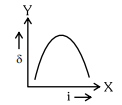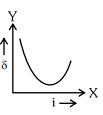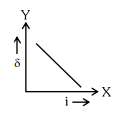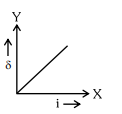# The expected graphical representation of the variation of angle of deviation`
Question:

The expected graphical representation of the variation of angle of deviation ' $\delta$ ' with angle of incidence ' $i$ ' in a prism is :

1.2.3.4.Correct Option: , 2

Solution:

Standard graph between angle of deviation and incident angle.